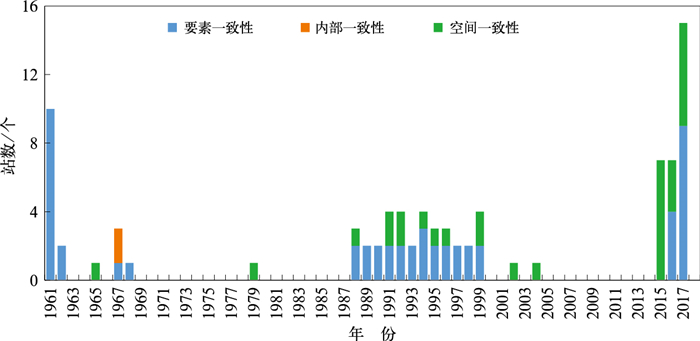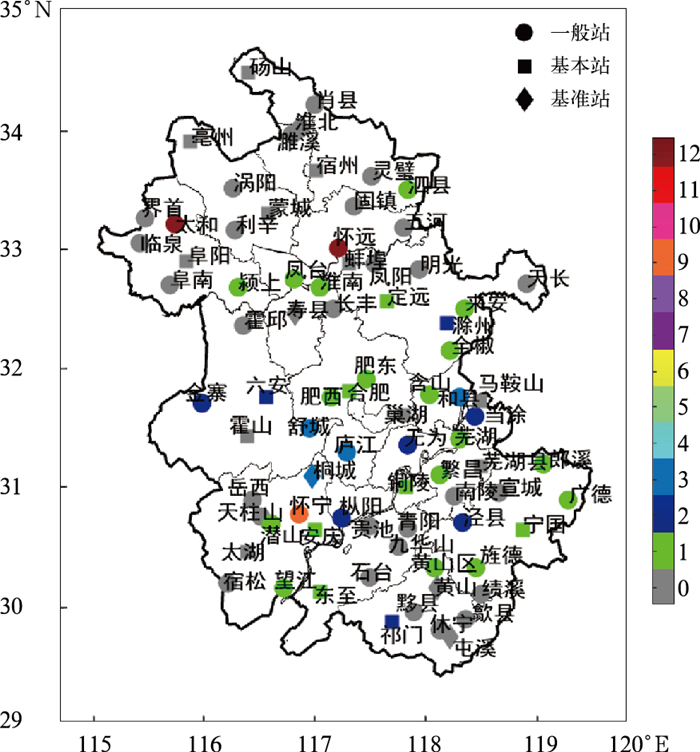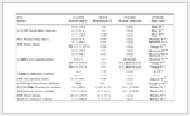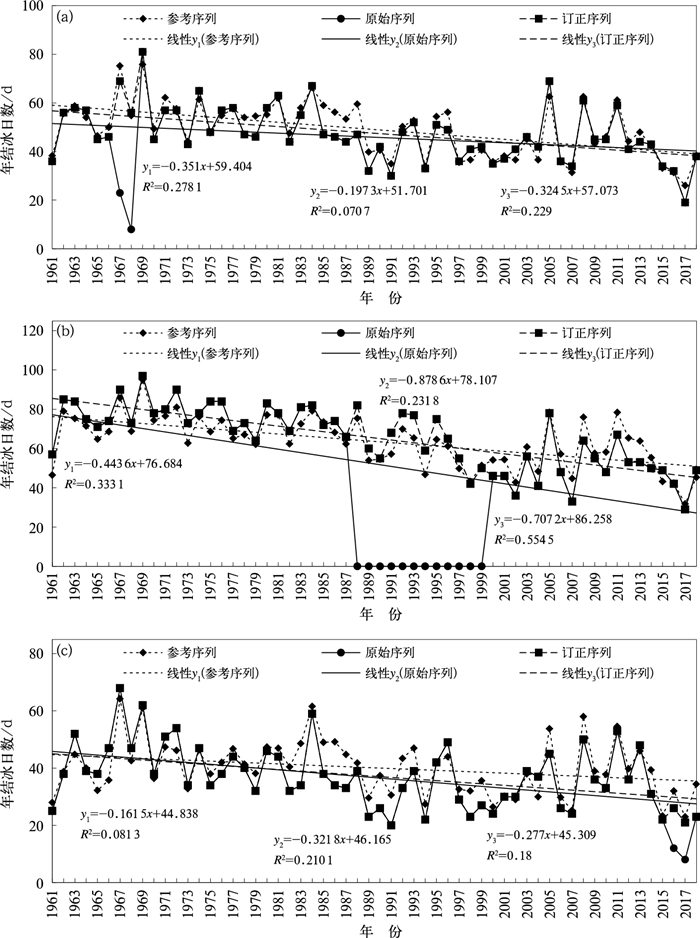﻿ 基于Bayes判别法的结冰现象资料序列订正
 快速检索 中文标题 英文标题 作者中文名 作者英文名 单位中文名 单位英文名 中文关键词 英文关键词 中文摘要 英文摘要 基金项目 全文
 气象2021, Vol. 47Issue (9): 1113-1121.  DOI: 10.7519/j.issn.1000-0526.2021.09.007### 引用本文 [复制中英文]

[复制中文]
WEN Huayang, ZHU Hualiang, MA Wenzhou, et al, 2021. Data Sequence Correction of Icing Weather Phenomena Based on Bayes Discriminant Method[J]. Meteorological Monthly, 47(9): 1113-1121. DOI: 10.7519/j.issn.1000-0526.2021.09.007.
[复制英文]

### 文章历史

2020年5月8日收稿
2021年7月16日收修定稿

1. 安徽省气象信息中心，合肥 230031
2. 合肥工业大学，合肥 230009

Data Sequence Correction of Icing Weather Phenomena Based on Bayes Discriminant Method
, ZHU Hualiang1, MA Wenzhou2, QIU Kangjun1, ZHANG Miaomiao1, LIU Zhuang2
1. Anhui Meteorological Information Centre, Hefei 230031;
2. Hefei University of Technology, Hefei 230009
Abstract: Using the methods of element consistency, internal consistency and spatial consistency, the data qualities of 81 national surface meteorological stations in Anhui Province from 1961 to 2018 are controlled. The Bayes discriminant model is trained based on the normal year data after quality control, and the icing data of abnormal year are corrected by the model. The results show that total 84 years' annual icing days from 38 stations in Anhui Province are abnormal, mainly concentrated in 1961-1970, 1988-1999 and 2015-2017, and fail to pass the quality control. The main reasons for these phenomena are the simplification of historical observation tasks of some stations, the adjustment of meteorological station classification, the reform of ground meteorological observation operation and so on. Then, by using the Bayes discriminant method, several discrimination models of icing weather phenomena are constructed. After verification, it is found that Model 1 and Model 3 have higher recognition accuracy, hit rate and TS score but lower false alarm rate. Considering the simplicity of calculation, Model 1 is selected to correct the icing data of abnormal years day by day. In addition, through the comparison of the icing weather phenomena correction results of Lu'an, Taihe and Wuwei Stations in abnormal years, we find that the model based on Bayes discriminant method is more reasonable for the correction of the icing weather phenomena caused by different reasons in different time periods, and the variation trend of annual icing days after correction is more in line with the actual situation, which shows that the Bayes discrimination model is reasonable and feasible to correct the icing weather phenomena.
Key words: icing weather phenomenon    sequence correction    Bayes discriminant method

1 资料与方法 1.1 资料与处理

1.2 研究方法

(1) 计算训练样本集中日结冰现象不发生和发生的概率分别为P0P1

(2) 假设某日的相关气象要素观测值X =(x1x2, …, xn)′，其中x1, x2, …, xn表示日最低气温、日最低地表温度等。根据训练样本集计算该日结冰现象不发生和发生情况下观测到相应气象要素值X的概率P(X /Yi), i=0, 1，其中Y0代表日结冰现象不发生，Y1代表日结冰现象发生。

(3) 根据Bayes定理，计算在相应气象要素观测值X条件下该日结冰现象不发生或发生的概率P(Yi/ X)：

 $\begin{array}{l} P\left({{Y_i}/{\boldsymbol{X}}} \right) = \frac{{P\left({{\boldsymbol{X}}/{Y_i}} \right){P_i}}}{{P\left({{\boldsymbol{X}}/{Y_i}} \right){P_0} + P\left({{\boldsymbol{X}}/{Y_1}} \right){P_1}}}\\ \;\;\;\;\;\;\;\;\;\;\;\;\;\;\;\;\;\;\;\;\;\;\;\;\;i = 0, 1 \end{array}$ (1)

$P\left({{Y_0}/{\boldsymbol{X}}} \right) = \mathop {\max }\limits_{i \in \left\{ {0, 1} \right\}} P\left({{Y_i}/{\boldsymbol{X}}} \right)$，即结冰现象不发生的概率大于发生的概率，则认为该日无结冰现象；否则，认为该日有结冰现象。假设结冰未发生条件下气象要素的协方差矩阵和结冰发生条件下气象要素的协方差矩阵相同，且都服从多元正态分布N(μi, Σ)，则有：

 $\left\{ \begin{array}{l} P\left({{\boldsymbol{X}}/{Y_0}} \right) = {\left({2\pi } \right)^{ - n/2}}{\left| {\mathit{\pmb{\Sigma}}} \right|^{ - 1/2}} \cdot \\ \;\;\;\;\;\;\;\;\;\;\;\;\;\;\;\exp \left[ { - \frac{1}{2}\left({{\boldsymbol{X}} - {{\boldsymbol{\mu}} _0}} \right)'{{\mathit{\pmb{\Sigma}}} ^{ - 1}}\left({{\boldsymbol{X}} - {{\boldsymbol{\mu}} _0}} \right)} \right]\\ P\left({{\boldsymbol{X}}/{Y_1}} \right) = {\left({2\pi } \right)^{ - n/2}}{\left| {\mathit{\pmb{\Sigma}}} \right|^{ - 1/2}} \cdot \\ \;\;\;\;\;\;\;\;\;\;\;\;\;\;\;\exp \left[ { - \frac{1}{2}\left({{\boldsymbol{X}} - {{\boldsymbol{\mu}} _1}} \right)'{{\mathit{\pmb{\Sigma}}} ^{ - 1}}\left({{\boldsymbol{X}} - {{\boldsymbol{\mu}} _1}} \right)} \right] \end{array} \right.$ (2)

 $\begin{array}{l} \mathop {\max }\limits_{i \in \left\{ {0, 1} \right\}} P\left({{Y_i}/{\boldsymbol{X}}} \right) \Leftrightarrow \mathop {\max }\limits_{i \in \left\{ {0, 1} \right\}} \left[ {P\left({{\boldsymbol{X}}/{Y_i}} \right){P_i}} \right]\\ \;\;\;\;\;\;\; \Leftrightarrow \mathop {\max }\limits_{i \in \left\{ {0, 1} \right\}} \ln \left[ {P\left({{\boldsymbol{X}}/{Y_i}} \right){P_i}} \right] \end{array}$

 $\begin{array}{l} \ln \left[ {P\left({{\boldsymbol{X}}/{Y_i}} \right){P_i}} \right] = \ln {P_i} - \frac{n}{2}\ln \left({2\pi } \right) - \\ \;\;\;\;\;\;\;\;\;\;\frac{1}{2}\ln \left| {\mathit{\pmb{\Sigma}}} \right| - \frac{1}{2}{\boldsymbol{X}}'{{\mathit{\pmb{\Sigma}}} ^{ - 1}}{\boldsymbol{X}} - \\ \;\;\;\;\;\;\;\;\;\;\frac{1}{2}{{\boldsymbol{\mu}} _i}'{\Sigma ^{ - 1}}{{\boldsymbol{\mu}} _i} + {\boldsymbol{X}}'{{\mathit{\pmb{\Sigma}}} ^{ - 1}}{{\boldsymbol{\mu}} _i} \end{array}$ (3)

 $\left\{ \begin{array}{l} {Y_0}\ln {P_0} + {a_{00}} + {a_{01}}{x_1} + \cdots + {a_{0n}}{x_n}\\ {Y_1}{\rm{ = }}\ln {P_1} + {a_{10}} + {a_{11}}{x_1} + \cdots + {a_{1n}}{x_n} \end{array} \right.$ (4)

 $\begin{array}{l} {a_{i0}} = - \frac{1}{2}{{\boldsymbol{\mu}} _i}'{{\mathit{\pmb{\Sigma}}} ^{ - 1}}{{\boldsymbol{\mu}} _i}\\ \;\;\;{a_{ij}} = \sum\limits_{l = 1}^n {{\sigma ^{jl}}{\mu _{il}}} \\ \;\;\;\;\;i = 0, 1, j = 1, 2, \cdots, n \end{array}$

Y0>Y1，认为该日无结冰现象；反之，认为该日有结冰现象。根据每日相关气象要素的观测值(日最低气温、日最低地表温度等)，利用式(4)可以完成逐日结冰现象的订正。

1.3 Bayes判识模型评价指标

 $PC = \frac{{TP + TN}}{{TN + FN + FP + TP}} \times 100\%$ (5)
 $POD = \frac{{TP}}{{FN + TP}} \times 100\%$ (6)
 $FAR = \frac{{TP}}{{FN + TP}} \times 100\%$ (7)
 $TS = \frac{{TP}}{{FP + FN + TP}} \times 100\%$ (8)

2 数据质量控制检查结果分析

 $\hat y = 3.55 + 0.965x$ (9)图 1 1961—2018年安徽省年结冰日数异常台站数逐年分布 Fig. 1 Distribution of stations with abnormal annual icing days in Anhui Province from 1961 to 2018图 2 1961—2018年安徽省各台站累计异常年数分布(单位：a) Fig. 2 Distribution of cumulative abnormal years of stations in Anhui Province from 1961 to 2018 (unit: a)
3 结冰现象资料序列订正 3.1 Bayes判别模型建立

 $\left\{ \begin{array}{l} {Y_0} = 0.6319 + 0.2726{T_{\min }}\\ {Y_1} = 0.5670 - 0.1496{T_{\min }} \end{array} \right.$ (10)

 $\left\{ \begin{array}{l} {Y_0} = 1.1136 + 0.1546{D_{\min }}\\ {Y_1} = 0.3349 - 0.1965{D_{\min }} \end{array} \right.$ (11)

 $\left\{ \begin{array}{l} {Y_0} = 23.5844 + 3.6126{T_{\min }} + 0.7133{D_{\min }}\\ {Y_1} = 18.3872 - 6.6013{T_{\min }} - 1.3021{D_{\min }} \end{array} \right.$ (12)表 1 基于验证数据集的各模型判别效果 Table 1 Discrimination effect of each model based on validation data set
3.2 基于Bayes判别模型的结冰序列订正表 2 异常站点年结冰日数的质量控制结果 Table 2 The quality control results of revised annual icing days at abnormal stations
3.3 订正结果对比图 3 1961—2018年六安站(a)、太和站(b)和无为站(c)年结冰日数序列 Fig. 3 Annual icing days series of Lu'an Station (a), Taihe Station (b) and Wuwei Station (c) from 1961 to 2018

4 结论与讨论

(1) 本研究采用要素一致性、内部一致性、空间一致性等方法对安徽省81个台站1961—2018年年结冰日数序列进行更细致的质量控制，与余予等(2018)中质量控制方法相比，本研究所采用的方法更为严格，主要体现在：①本研究内部一致性检查时采用3倍标准差，而余予等(2018)采用5倍标准差；②本研究采用了空间一致性检查，而余予等(2018)未进行空间一致性检查。

(2) 年结冰日数质量控制结果表明，安徽省共有38个台站累计84年的年结冰日数质量控制检查异常，年结冰日数异常年份主要集中在1961—1970、1988—1999和2015—2017年，造成年结冰日数异常的原因有部分台站历史观测任务简化、气象台站分类调整以及地面气象观测业务改革等。余予等(2018)对安徽省1981年之前的年结冰日数质量控制发现，共有1站累计1年未记录结冰现象，有8站存在1~2年的年结冰日数显著偏低。从图 1可以看出，本研究发现的1981年之前的年结冰日数异常站数和累计年数要明显多于余予等(2018)的质量控制结果，这也佐证了本研究质量控制标准比余予等(2018)的质量控制标准更为严格。

(3) 针对质量控制后的正确年份结冰现象数据集，采用Bayes判别法建立模型1、模型2和模型3。经检验发现，模型1和模型3各项评价指标均较好。考虑计算的简便性，选用模型1对质量控制检查异常年份结冰数据进行逐日订正。通过订正结果对比发现，基于Bayes判别法的结冰现象判别模型，对不同时间段内，不同原因造成的结冰现象观测记录异常的订正均较为合理，订正后的年结冰日数变化趋势更符合实际情况，表明采用Bayes判别模型订正结冰现象是合理、可行的。

(4) 本研究发现部分台站历史观测任务简化、气象台站分类调整、地面气象观测业务改革等引发了安徽省部分台站的年结冰日数异常问题，这些问题势必在全国其他地区同样存在，余予等(2018)仅对1981年前的年结冰日数进行质量控制，就发现全国1954—1979年共有662个台站累计6 328年实际未观测和记录结冰现象，1981年前有839个台站累计1 453年存在明显漏记结冰现象情况。因此，在使用全国结冰资料进行气候变化分析时，还需进行有效的质量控制和序列订正。本研究所用方法对全国范围内的结冰资料的质量控制和订正提供了一种参考，但其质量控制和订正效果还有待于进一步探讨。

 白永清, 陈城, 何明琼, 2016. 利用Logistic回归方法的高速桥(路)面结冰风险等级预警模型[J]. 气象科技, 44(2): 336-340. Bai Y Q, Chen C, He M Q, 2016. Warning model of risk degree for bridge/road surface icing of expressway based on Logistic regression[J]. Meteor Sci Technol, 44(2): 336-340 (in Chinese). DOI:10.3969/j.issn.1671-6345.2016.02.026 丁国香, 刘承晓, 周建平, 等, 2018. 气象条件对安徽高速公路运营的影响[J]. 干旱气象, 36(5): 858-863. Ding G X, Liu C X, Zhou J P, et al, 2018. Impact of meteorological conditions on expressway operation in Anhui Province[J]. J Arid Meteor, 36(5): 858-863 (in Chinese). 范邵华, 余予, 鞠晓慧, 等, 2018. 气象资料数字化进展及应用[J]. 气象科技进展, 8(1): 64-70, 78. Fan S H, Yu Y, Ju X H, et al, 2018. A review for the progesses and applications of historical meteorological data rescuing and digitizing over China[J]. Adv Meteor Sci Technol, 8(1): 64-70, 78 (in Chinese). DOI:10.3969/j.issn.2095-1973.2018.01.007 华连生, 温华洋, 朱华亮, 等, 2015. 基于Bayes判别法的霜生自动化观测模型探讨[J]. 气象, 41(8): 964-969. Hua L S, Wen H Y, Zhu H L, et al, 2015. Automated observation model for frost based on Bayes discriminant method[J]. Meteor Mon, 41(8): 964-969 (in Chinese). 江益, 王立俊, 羊清雯, 等, 2018. 南海区域站气象资料质量控制方法改进[J]. 气象科技, 46(3): 474-478, 496. Jiang Y, Wang L J, Yang Q W, et al, 2018. Improvement of quality control method for real time meteorological data from regional automatic stations on South China Sea[J]. Meteor Sci Technol, 46(3): 474-478, 496 (in Chinese). 李刚, 马继望, 梁湘三, 2020. 2008年1月中国南方低温雨雪期间异常阻塞高压事件的多尺度动力过程分析[J]. 气象学报, 78(1): 18-32. Li G, Ma J W, Liang X S, 2020. A study of the multiscale dynamical processes underlying the blocking high that caused the January 2008 freezing rain and snow storm in southern China[J]. Acta Meteor Sin, 78(1): 18-32 (in Chinese). 廖捷, 周自江, 2018. 全球常规气象观测资料质量控制研究进展与展望[J]. 气象科技进展, 8(1): 56-63. Liao J, Zhou Z J, 2018. Quality control of the global conventional meteorological observations: progresses and prospects[J]. Adv Meteor Sci Technol, 8(1): 56-63 (in Chinese). DOI:10.3969/j.issn.2095-1973.2018.01.006 刘洪兰, 张俊国, 阙龙凯, 等, 2014. 基于BP神经网络的张掖国家湿地公园水域结冰厚度预报模型[J]. 高原气象, 33(3): 832-837. Liu H L, Zhang J G, Que L K, et al, 2014. Forecasting model for ice thickness in Zhangye National Wetland Park watershed based on BP neural network[J]. Plateau Meteor, 33(3): 832-837 (in Chinese). 刘小宁, 任芝花, 2005. 地面气象资料质量控制方法研究概述[J]. 气象科技, 33(3): 199-203. Liu X N, Ren Z H, 2005. Progress in quality control of surface meteorological data[J]. Meteor Sci Technol, 33(3): 199-203 (in Chinese). DOI:10.3969/j.issn.1671-6345.2005.03.002 马树庆, 袭祝香, 马力文, 等, 2015. 北方水稻低温冷害指标持续适用性检验与比较[J]. 气象, 41(6): 778-785. Ma S Q, Xi Z X, Ma L W, et al, 2015. Test and comparison of sustainable suitability of meteorological indicators for rice cold damages in the North of China[J]. Meteor Mon, 41(6): 778-785 (in Chinese). 闵锦忠, 王晨珏, 贾瑞怡, 2018. 苏皖地面自动站资料的质量控制及结果分析[J]. 大气科学学报, 41(5): 637-646. Min J Z, Wang C J, Jia R Y, 2018. Quality control and result analysis for surface AWS data in Jiangsu and Anhui Provinces[J]. Trans Atmos Sci, 41(5): 637-646 (in Chinese). 宁惠芳, 张志富, 范邵华, 2014. 浅析《地面气象观测规范》的演变及对资料质量控制的影响[J]. 青海气象, (3): 53-56. Ning H F, Zhang Z F, Fan S H, 2014. Analysis of the evolution of "ground meteorological observation standard" and its influence on data quality control[J]. J Qinghai Meteor, (3): 53-56 (in Chinese). 蒲晓虎, 张春波, 马舒庆, 等, 2014. 地面气象观测中结冰自动化观测的研究[J]. 气象, 40(2): 234-237. Pu X H, Zhang C B, Ma S Q, et al, 2014. Research on icing automation observation in ground meteorological observation[J]. Meteor Mon, 40(2): 234-237 (in Chinese). 任芝花, 熊安元, 邹凤玲, 2007. 中国地面月气候资料质量控制方法的研究[J]. 应用气象学报, 18(4): 516-523. Ren Z H, Xiong A Y, Zou F L, 2007. The quality control of surface monthly climate data in China[J]. J Appl Meteor Sci, 18(4): 516-523 (in Chinese). DOI:10.3969/j.issn.1001-7313.2007.04.012 任芝花, 张志富, 孙超, 等, 2015. 全国自动气象站实时观测资料三级质量控制系统研制[J]. 气象, 41(10): 1268-1277. Ren Z H, Zhang Z F, Sun C, et al, 2015. Development of three-step quality control system of real-time observation data from AWS in China[J]. Meteor Mon, 41(10): 1268-1277 (in Chinese). DOI:10.7519/j.issn.1000-0526.2015.10.010 舒斯, 熊守权, 陈英英, 等, 2019. 湖北省高速公路道路结冰预警模型[J]. 气象, 45(11): 1589-1599. Shu S, Xiong S Q, Chen Y Y, et al, 2019. Warning model of road surface icing of expressway in Hubei Province[J]. Meteor Mon, 45(11): 1589-1599 (in Chinese). DOI:10.7519/j.issn.1000-0526.2019.11.009 唐熠, 周秀华, 郑传新, 等, 2019. 广西重大低温雨雪冰冻过程500 hPa信号场异常特征分析[J]. 气象, 45(10): 1446-1456. Tang Y, Zhou X H, Zheng C X, et al, 2019. Analysis of abnormal characteristics at 500 hPa signal field in major cryogenic freezing rain and snow processes in Guangxi[J]. Meteor Mon, 45(10): 1446-1456 (in Chinese). DOI:10.7519/j.issn.1000-0526.2019.10.011 陶士伟, 仲跻芹, 徐枝芳, 等, 2009. 地面自动站资料质量控制方案及应用[J]. 高原气象, 28(5): 1202-1209. Tao S W, Zhong J Q, Xu Z F, et al, 2009. Quality control schemes and its application to automatic surface weather observation system[J]. Plateau Meteor, 28(5): 1202-1209 (in Chinese). 王斌会, 2011. 多元统计分析及R语言建模: 第2版[M]. 广州: 暨南大学出版社: 113-153. Wang B H, 2011. Multivariate Statistical Analysis and Modeling for R Language: 2nd ed.[M]. Guangzhou: Jinan University Press: 113-153 (in Chinese). 吴增祥, 2006. 中国地面气象台站(1950—2004)沿革情况概述[C]//中国气象学会2006年年会"气象史志研究进展"分会场论文集. 成都: 中国气象学会: 87-97. Wu Z X, 2006. An overview of the evolution of China's surface meteorological stations(1950-2004)[C]//Proceedings of the "Research Progress of Meteorological History" branch of the 2006 Annual Meeting of China Meteorological Society. Chengdu: China Meteorological Society: 87-97(in Chinese). 余予, 任芝花, 孟晓艳, 2018. 中国结冰现象序列的建立及气候变化分析[J]. 高原气象, 37(2): 553-559. Yu Y, Ren Z H, Meng X Y, 2018. Construction and climate variation analysis of icing weather phenomenon series over China[J]. Plateau Meteor, 37(2): 553-559 (in Chinese). 张庆奎, 项阳, 吉振明, 等, 2020. 近55年安徽省结冰现象气候特征及趋势分析[J]. 自然灾害学报, 29(6): 218-226. Zhang Q K, Xiang Y, Ji Z M, et al, 2020. Analysis of climatic characteristics and trends for icing weather phenomenon in Anhui Province in recent 55 years[J]. J Nat Dis, 29(6): 218-226 (in Chinese). 张志富, 希爽, 余予, 等, 2015. 1961—2012年中国5类主要冰冻天气的气候及变化特征[J]. 冰川冻土, 37(6): 1435-1442. Zhang Z F, Xi S, Yu Y, et al, 2015. Climatic characteristics and variations of the gelivation weathers in China during 1961-2011[J]. J Glaciol Geocryol, 37(6): 1435-1442 (in Chinese). 中国气象局, 1961. 地面气象观测规范[M]. 北京: 气象出版社: 83-98. China Meteorological Administration, 1961. Specification for Ground Meteorological Observation[M]. Beijing: China Meteorological Press: 83-98 (in Chinese). 中国气象局, 2003. 地面气象观测规范[M]. 北京: 气象出版社: 21-27. China Meteorological Administration, 2003. Specification for Ground Meteorological Observation[M]. Beijing: China Meteorological Press: 21-27 (in Chinese). 中国气象局综合观测司, 2013. (2013-12-05) [2014-01-08]. 关于做好全国地面气象观测业务调整工作的通知: 气测函321号[A/OL]. https://wenku.baidu.com/view/55b1110bf01-dc281e53af0c6.html. Department of Integrated Observations, China Meteorological Administration, 2013. (2013-12-05) [2014-01-08]. Notice on the adjustment of national surface meteorological observation[A/OL]. https://wenku.baidu.com/view/55b1110bf01dc281e53af0c6.html (in Chinese). Toms B A, Basara J B, Hong Y, 2017. Usage of existing meteorological data networks for parameterized road ice formation modeling[J]. J Appl Meteor Climatol, 56(7): 1959-1976. DOI:10.1175/JAMC-D-16-0199.1 Wang Y G, Zhou Z J, Zhang Q, et al, 2008. Main meteorological disasters and their impacts on the economic and societal developments in China[J]. Int J Risk Assess Manage, 8(4): 384-394. DOI:10.1504/IJRAM.2008.019024